# Physics GRE 5. Quantum Mechanics and Atomic Physics EssayViola Marenco
question

5.1 Average Value of Operator, “A”, on state psi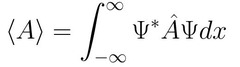question

5.2 Position-Probability Normalization of state psi

question

5.3 Property of Hermitian Operator “Q” acting on states f and g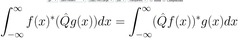question

5.4 Position and Momentum Operators

question

5.5 Probability of Obtaining nth eigenvalue

question

5.6 Expectation (Average) Value

question

5.7 Inner product of and a and b (Bracket Notation)

question

5.8 Bracket Notation Inner product commutative property

question

5.9 Hermitian Conjugate Condition for inner product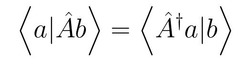question

5.10 f(x), inner product relation for continuum states

question

5.11 inner product relation for states f,g with continuous position locations x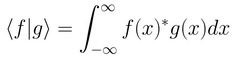question

5.12 Schrodinger Equation

question

5.13 Hamiltonian Operator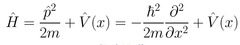question

5.14 Schrodinger, Eigenvalue Equation

question

5.15 Position, Momentum commutation relation

question

5.16 Uncertainty relation of operators A and B based off of their commutator

question

5.17 Statistical variance/ Uncertainty

question

5.18 Uncertainty Product for position and momentum

question

5.19 Order of Magnitude estimate of position and momentum uncertainty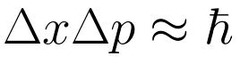question

5.20 Energy and Time order of magnitude momentum and position uncertainty

question

5.21 One Dimensional Harmonic Oscillator Hamiltonian

question

5.22 One dimensional Harmonic Oscillator Hamiltonian using raising and lowering operators

question

5.23 Commutation relation of lowering and raising operators

question

5.24 One Dimensional Harmonic Oscillator Energy Eigenvalues

question

5.25 Virial’s Theorem for Harmonic Oscillator

question

5.26 Three Dimensional Harmonic Oscillator Energy Eigenvalues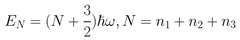question

5.27 Eigenfunctions and Energy Eigenvalues of a free particle

question

5.28 De Broglie Formula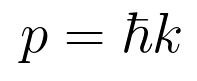question

5.29 Einstein’s relation (energy of a free particle)

question

5.30 General Hamiltonian in Three Dimensions

question

5.31 3 Dimensional, position-momentum commutation along same direction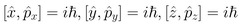question

5.32 3 Dimensional, position-momentum commutation along different directions

question

5.33 Radial Wave function Normalization conditions

question

5.34 x component of orbital angular momentum

question

5.35 y component of orbital angular momentum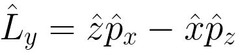question

5.36 z component of orbital angular momentum

question

5.37 Commutator relation of orthogonal components of orbital angular momentum

question

5.38 Total orbital angular momentum

question

5.39 Z component of angular momentum acting on a spherical harmonic state

question

5.40 Total orbital angular momentum acting on a spherical harmonic state

question

5.41 Allowed values of m (magnetic moment) in terms of l (orbital angular momentum)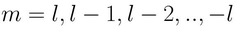question

5.42 Orthonormaility of Spherical Harmonics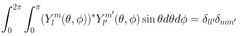question

5.43 Hamiltonian of Hydrogen Atom

question

question

5.45 Un-normalized Ground state of Hydrogen Atom

question

5.46 Hydrogen Ground State Energy

question

5.47 nth Energy Eigenvalues of Hydrogen considering quantum number n only

question

5.48 fine structure constant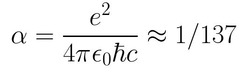question

5.49 spin up and down along x in the z basis

question

5.50 spin up and down along y in z basis

question

5.51 Raising and Lowering Operators for spin 1/2 in terms of spin along x and y

question

5.54 Adding spins s and s’

question

5.55 Adding z component of spin quantum number m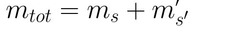question

5.56 Singlet State a system of 2 spin 1/2 particles

question

5.57 Perturbation Theory, 1st order energy Shift

question

5.58 Perturbation Theory, 2nd order energy shift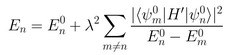question

5.59 Eigenvalues of Spin-Orbit Operator, fine structure perturbation

question

5.60 Change in Hamiltonian from Stark Effect